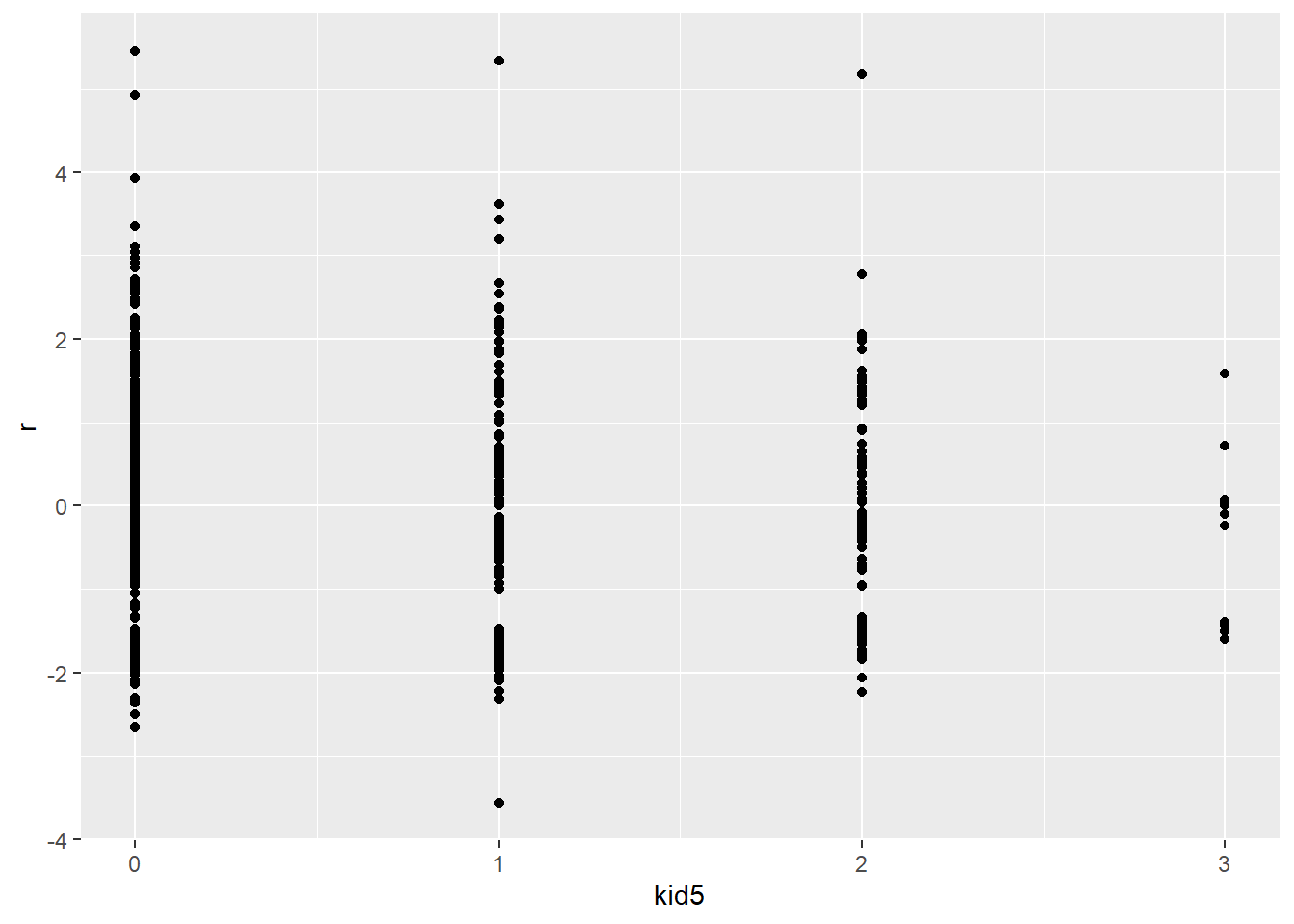# Deviance in a generalized linear model

## 2021-09-20

Deviance is a measure computed for generalized linear models that can help you decide between two competing models. It is somewhat analagous to residual sums of squares in linear regression.

suppressMessages(suppressWarnings(library(broom)))
suppressMessages(suppressWarnings(library(dplyr)))
suppressMessages(suppressWarnings(library(foreign)))
suppressMessages(suppressWarnings(library(ggplot2)))
suppressMessages(suppressWarnings(library(magrittr)))

### Maximum likelihood equations for a generalized linear model

The maximum likelihood equations are pretty easy to compute.

$$L(y, \theta)=\prod_i f(y, \theta)$$

$$ln L(y, \theta)=\sum_i ln(f(y, \theta)$$

If we write the distribution in the canonical form

$$ln f(y, \theta)=\frac{\theta y+b(\theta)}{a(\phi)}+c(y, \phi)$$

which means that

$$ln L(y, \theta)=\sum_i \frac{\theta y}{a(\phi)} + \sum_i \frac{b(\theta)}{a{\phi}}+\sum_i c(y_i, \theta)$$

For a Poisson regression model,

$$ln L(y, \theta)=\sum_i \theta y_i + \sum_i e^\theta - \sum_i ln(y_i!)$$

The deviance is defined as

$$D=-2(ln L(y, y) - ln L(y, \hat \theta))$$

which in the Poisson regression model equals

$$D=2(\sum_i y_i(y_i-\hat \theta)+e^{y_i}-e^{\hat \theta})$$

In terms of $$\hat \theta$$, this converts to

$$D=2 \sum_i (y_i ln(y_i/ \hat\theta)-(y_i-\hat\lambda))$$

You want the deviance to as small as possible. So any term inside this sum that is big represents a data value that is contributing a lot to lack of fit.

The individual terms in this sum are all positive, so we have to account for whether the lack of fit is due to underprediction or overprediction. So the deviance residual is defines as

$$sign(y_i-\hat\theta)\sqrt{2(y_i ln(y_i / \hat\theta)- (y_i-\hat\theta))}$$

### Computing deviance residuals in R

Francis Huang has a nice web page showing two datasets and how to analyze them using the Poisson regression model as well as several more sophisticated models. The first data set is available for anyone to download. The link I originally used no longer works.

fn <- "http://faculty.missouri.edu/huangf/data/poisson/articles.csv"
head(articles)

Here is a different link for the same file, used at German Rodriguez’s website. I’ve placed a copy on my website in case this link breaks. Also, this dataset is available with the pscl library of R under the name, bioChemists.

fn <- "http://www.stata-press.com/data/lf2/couart2.dta"
head(articles)
##   art   fem     mar kid5  phd ment
## 1   0   Men Married    0 2.52    7
## 2   0 Women  Single    0 2.05    6
## 3   0 Women  Single    0 3.75    6
## 4   0   Men Married    1 1.18    3
## 5   0 Women  Single    0 3.75   26
## 6   0 Women Married    2 3.59    2
fn <- "http://www.stata-press.com/data/lf2/couart2.dta"
head(articles)
##   art   fem     mar kid5  phd ment
## 1   0   Men Married    0 2.52    7
## 2   0 Women  Single    0 2.05    6
## 3   0 Women  Single    0 3.75    6
## 4   0   Men Married    1 1.18    3
## 5   0 Women  Single    0 3.75   26
## 6   0 Women Married    2 3.59    2

The variables are + gender (fem), + marital status (mar), + number of children (kid5), + prestige of graduate program (phd), + the number of articles published by the individual’s mentor (ment) + the number of articles published by the scientist (art: the outcome).

To fit a Poisson regression model in R, use the glm function with the argument, family=“poisson”.

pois1 <- glm(art ~ fem + ment + phd + mar + kid5, family=poisson, data = articles)
summary(pois1)
##
## Call:
## glm(formula = art ~ fem + ment + phd + mar + kid5, family = poisson,
##     data = articles)
##
## Deviance Residuals:
##     Min       1Q   Median       3Q      Max
## -3.5672  -1.5398  -0.3660   0.5722   5.4467
##
## Coefficients:
##              Estimate Std. Error z value Pr(>|z|)
## (Intercept)  0.304617   0.102981   2.958   0.0031 **
## femWomen    -0.224594   0.054613  -4.112 3.92e-05 ***
## ment         0.025543   0.002006  12.733  < 2e-16 ***
## phd          0.012823   0.026397   0.486   0.6271
## marMarried   0.155243   0.061374   2.529   0.0114 *
## kid5        -0.184883   0.040127  -4.607 4.08e-06 ***
## ---
## Signif. codes:  0 '***' 0.001 '**' 0.01 '*' 0.05 '.' 0.1 ' ' 1
##
## (Dispersion parameter for poisson family taken to be 1)
##
##     Null deviance: 1817.4  on 914  degrees of freedom
## Residual deviance: 1634.4  on 909  degrees of freedom
## AIC: 3314.1
##
## Number of Fisher Scoring iterations: 5
rd <- articles
rd$r <- resid(pois1, type="deviance") rd$p <- predict(pois1, type="response")
ggplot(rd, aes(r)) +
geom_histogram(bin_width=0.2)
## Warning: Ignoring unknown parameters: bin_width
## stat_bin() using bins = 30. Pick better value with binwidth.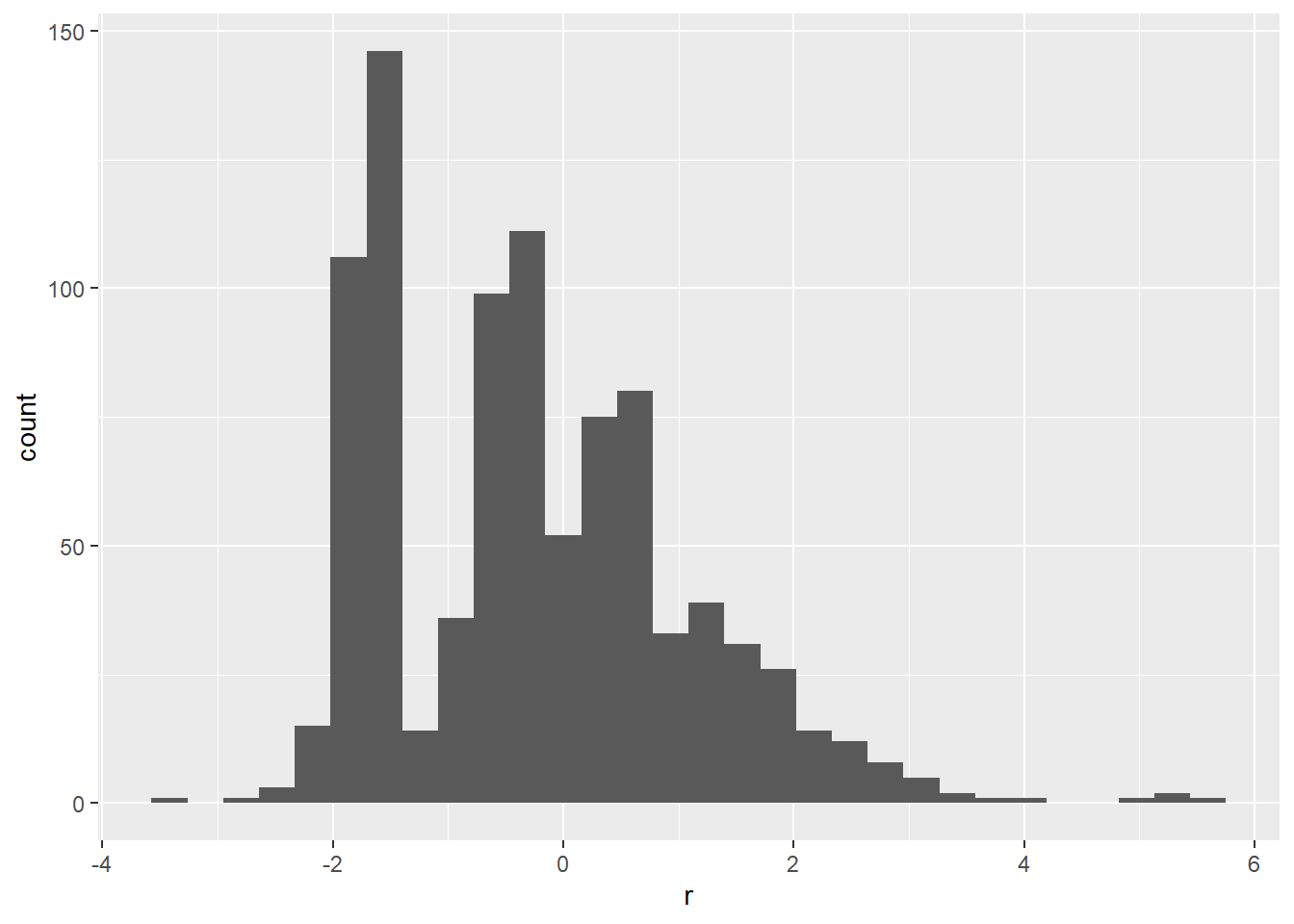ggplot(rd, aes(p, r)) +
geom_point()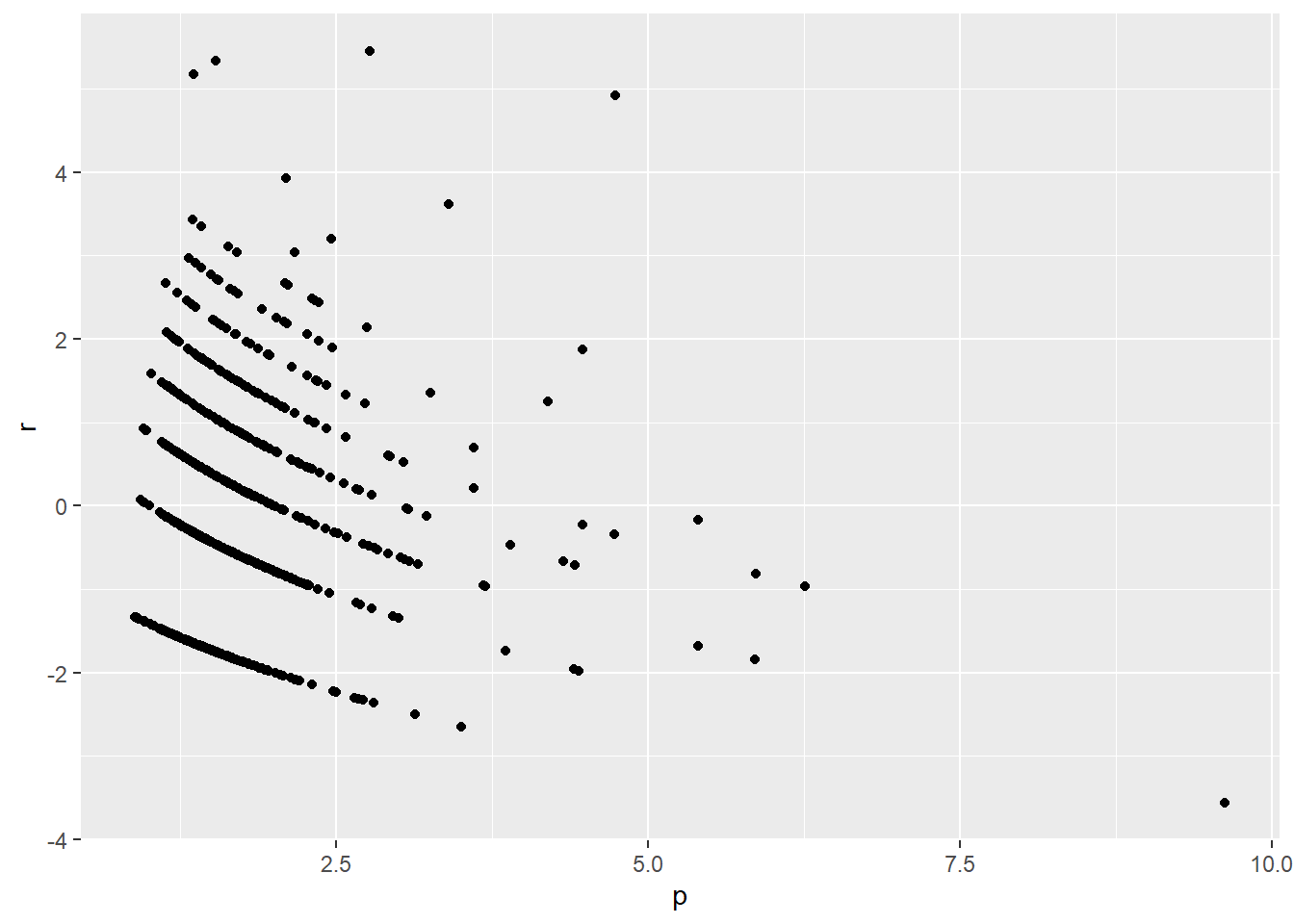ggplot(rd, aes(fem, r)) +
geom_point()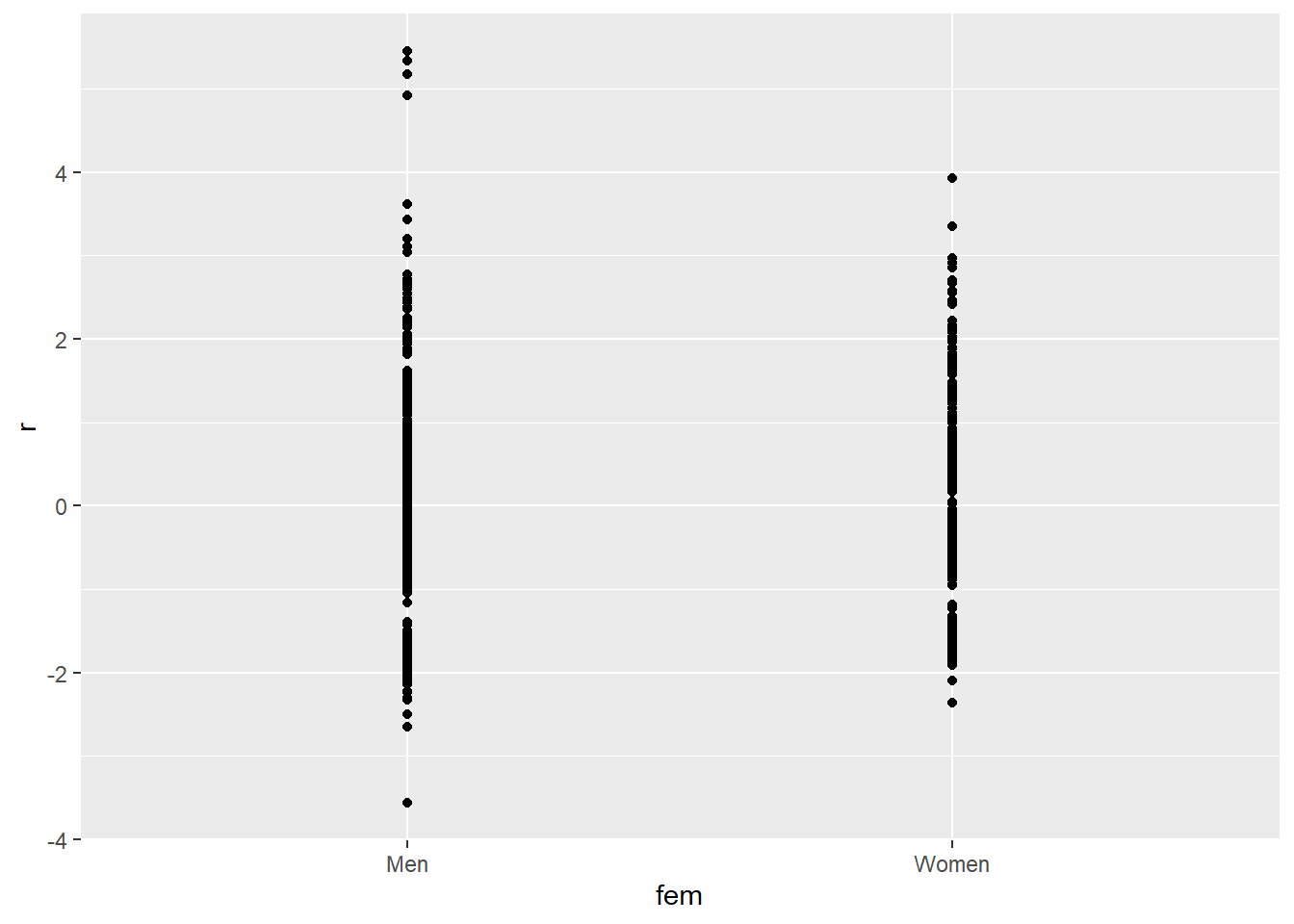ggplot(rd, aes(ment, r)) +
geom_point()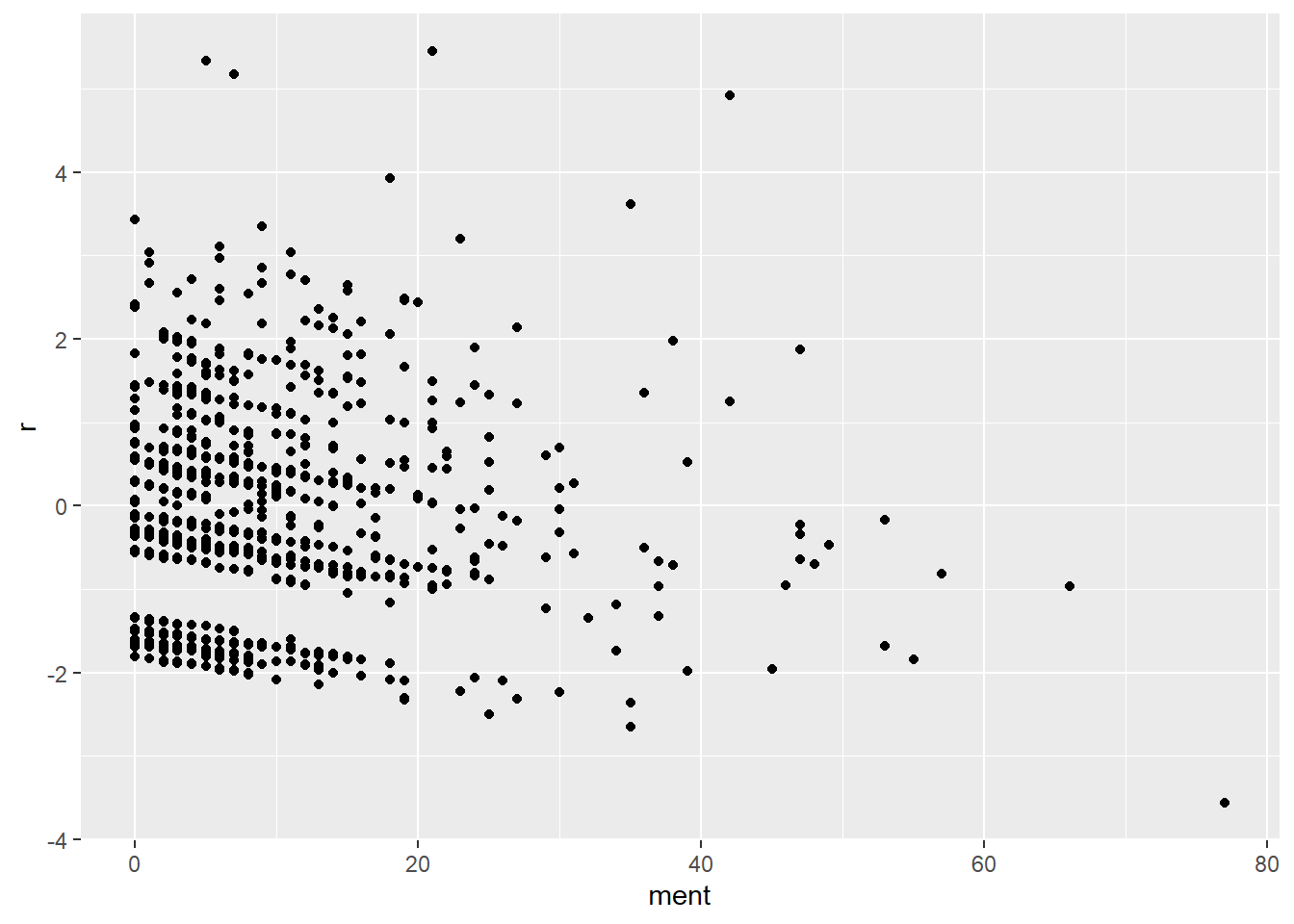ggplot(rd, aes(phd, r)) +
geom_point()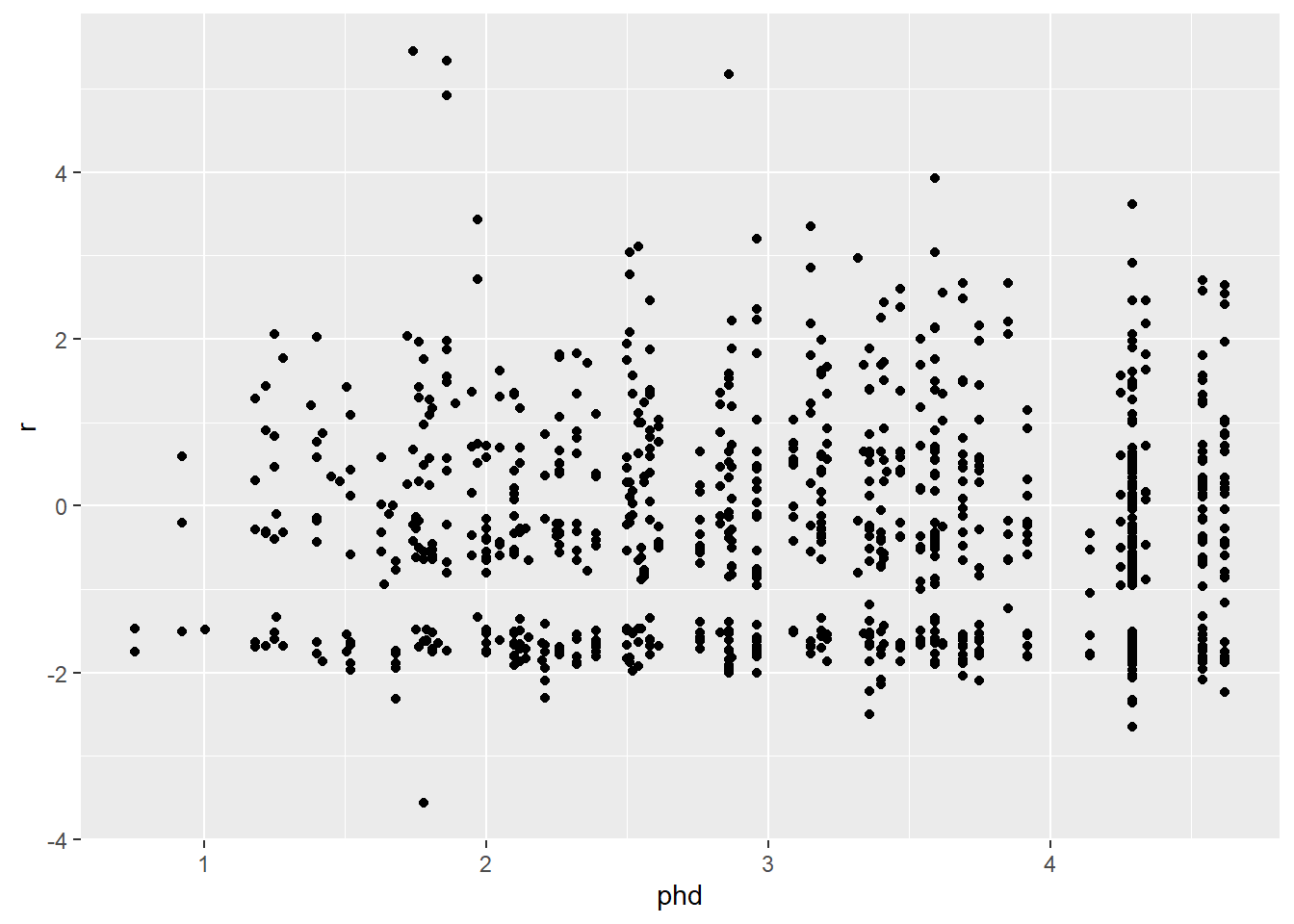ggplot(rd, aes(mar, r)) +
geom_point()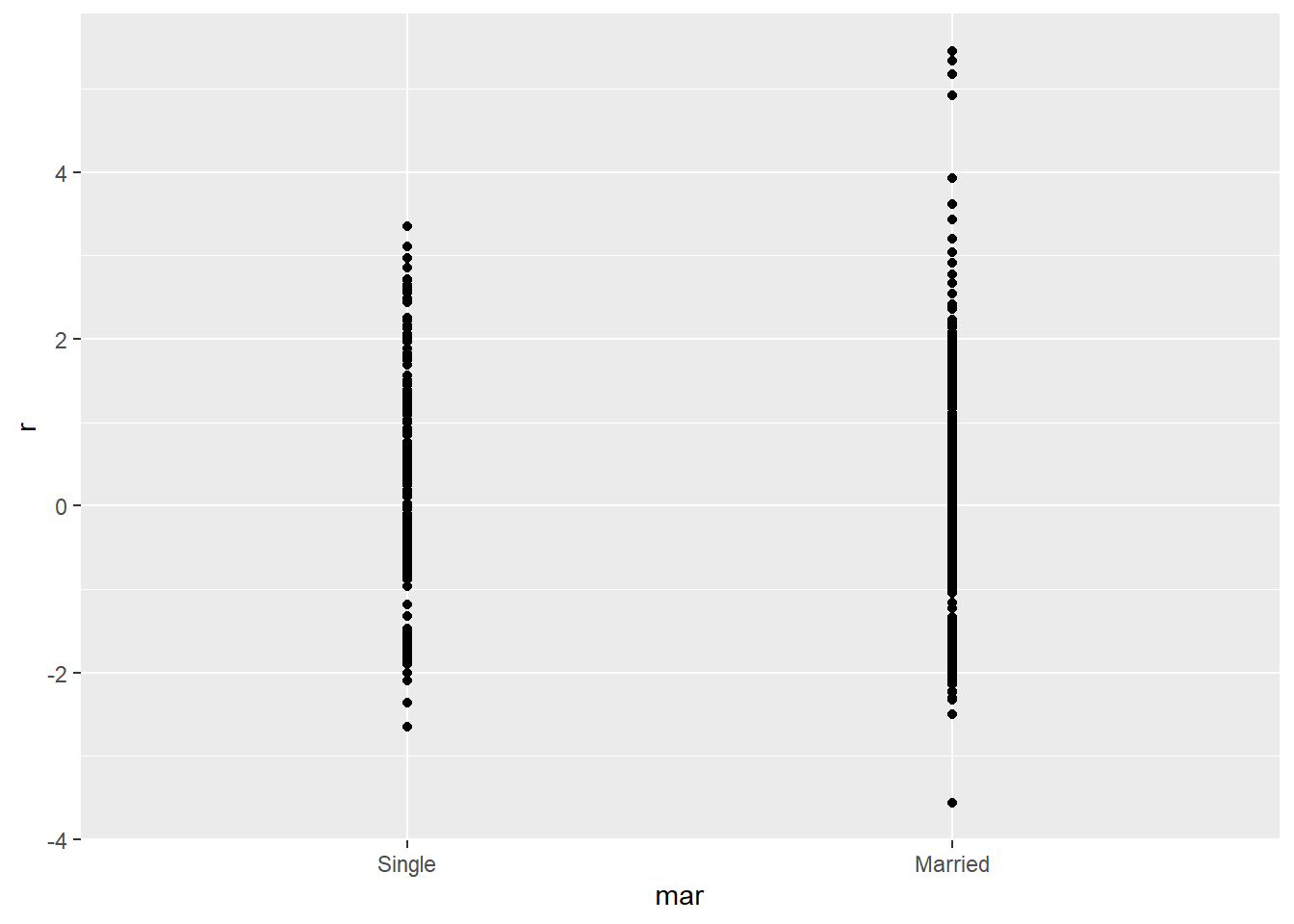ggplot(rd, aes(kid5, r)) +
geom_point()